# Civil Engineering - Strength of Materials

### Exercise :: Strength of Materials - Section 3

26.

For a simply supported beam carrying uniformly distributed load W on it entire length L, the maximum bending moment is

 A.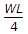B.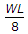C.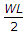D.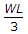E.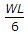Explanation:

No answer description available for this question. Let us discuss.

27.

Euler's formula states that the buckling load P for a column of length l, both ends hinged and whose least moment of inertia and modulus of elasticity of the material of the column are I and E respectively, is given by the relation

 A.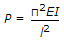B.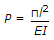C.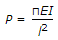D.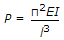E. P = πEl2.

Explanation:

No answer description available for this question. Let us discuss.

28.

The stiffness factor for a prismatic beam of length L and moment of inertia I, is

 A.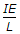B.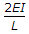C.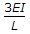D.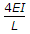E.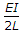Explanation:

No answer description available for this question. Let us discuss.

29.

The stress in the wall of a cylinder in a direction normal to its longitudinal axis, due to a force acting along the circumference, is known as

 A. yield stress B. longitudinal stress C. hoop stress D. circumferential stress E. ultimate stress.

Explanation:

No answer description available for this question. Let us discuss.

30.

In a simply supported beam (l + 2a) with equal overhangs (a) and carrying a uniformly distributed load over its entire length, B.M. at the middle point of the beam will be zero if

 A. l = 2a B. l = 4a C. l < 2a D. l > a E. l > 3a.